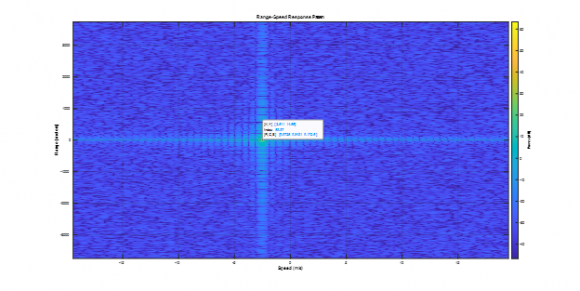## Range Doppler of an FMCW Radar.Started by 2 years ago370 views

Plotting the Range Doppler of an FMCW Radar developed for Vital Signs.

The FMCW radar with the following parameters was implemented:

%System parametersValue

%----------------------------------

%Operating frequency (GHz)77

%Maximum target range (m)15

%Range resolution (m)0.037

%Maximum target speed (km/h)30 for fall detection

%Sweep time (microseconds)50

%Sweep bandwidth (GHz)4

%Maximum beat frequency (MHz) 73

%Sample rate (GHz)4.050

A target was simulated with the following parameters(static radar)

dist = 14;

speed = 10*1000/3600; ;%2.77km/h

rcs = 100;

and the Range Doppler image plotted using the phased array functions.

RangeFFTLength = 2048;

DopplerFFTLength = 512;

rngdopresp = phased.RangeDopplerResponse('PropagationSpeed',c,...

'DopplerOutput','Speed','OperatingFrequency',fc,'SampleRate',fs,...

'RangeMethod','FFT','SweepSlope',sweep_slope,...

'RangeFFTLengthSource','Property','RangeFFTLength',RangeFFTLength,...

'DopplerFFTLengthSource','Property','DopplerFFTLength',DopplerFFTLength);

figure

plotResponse(rngdopresp,xr);The results are as expected.

Interested to get the same results implementing the 2DFFT manually.

For this purpose the following code was used:

Nr = RangeFFTLength;

Nd = DopplerFFTLength;

% 2D FFT using the FFT size for both dimensions.

sig_fft2 = fft2(xr,Nr,Nd);

% Taking just one side of signal from Range dimension.

sig_fft2 = sig_fft2(1:Nr/2,1:Nd);

sig_fft2 = fftshift (sig_fft2);

RDM = abs(sig_fft2);

RDM = 10*log10(RDM);

Yielding the following:The index of the range and velocity are: [518 229].

This yields the following values:

Range:

fb=fs/2;

range_max = c*fb/(2*sweep_slope);

distance = ((518-512)/512)*range_max

distance =

43.9453

This is 3 times larger then the correct value. Why?

Velocity:

Converting the index to the abs value using the following, yields correct value:

vel_max = lambda/(4*tm);

sp = ((293-256)/256)*vel_max

sp =

2.8155

Seems, however, that the velocity in this case is positive – why?

The code can be seen below: